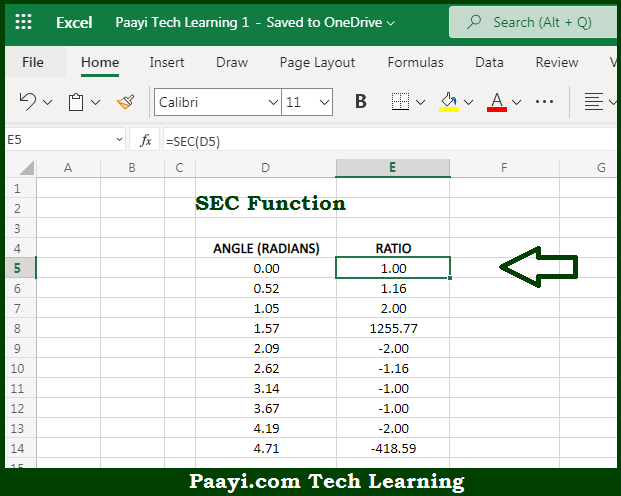# Learn How to Use Microsoft Excel SEC Function

Written by | 0 Comments | 531 Views

In this article, you will learn how to use the Microsoft Excel SEC function and its prime function in Microsoft Excel. You will also get to know the Microsoft Excel SEC function return value and syntax with the help of some examples.

Microsoft Excel SEC Function

The main purpose of the Microsoft Excel SEC function is to get the secant of the angle. That implies, with the help of the SEC function you can able to return the secant of an angle provided in radians. In geometry, the secant of a right triangle's angle is equal to the ratio of the length of its hypotenuse over the length of its adjacent side. So, with the help of the SEC function, you can able to get the secant of an angle provided in the radians.

Return Value of SEC Function

The return value will be the secant value.

Syntax of SEC Function

=SEC(number)

Where the arguments:

• number: This is the angle in radians of which you want to get the secant.

## How to Use Microsoft Excel SEC Function?So we know that Microsoft Excel SEC function you can able to get the secant of the angle. That implies, with the help of the SEC function you can able to return the secant of an angle provided in radians. In geometry, the secant of a right triangle's angle is equal to the ratio of the length of its hypotenuse over the length of its adjacent side. So, with the help of the SEC function, you can able to get the secant of an angle provided in the radians.